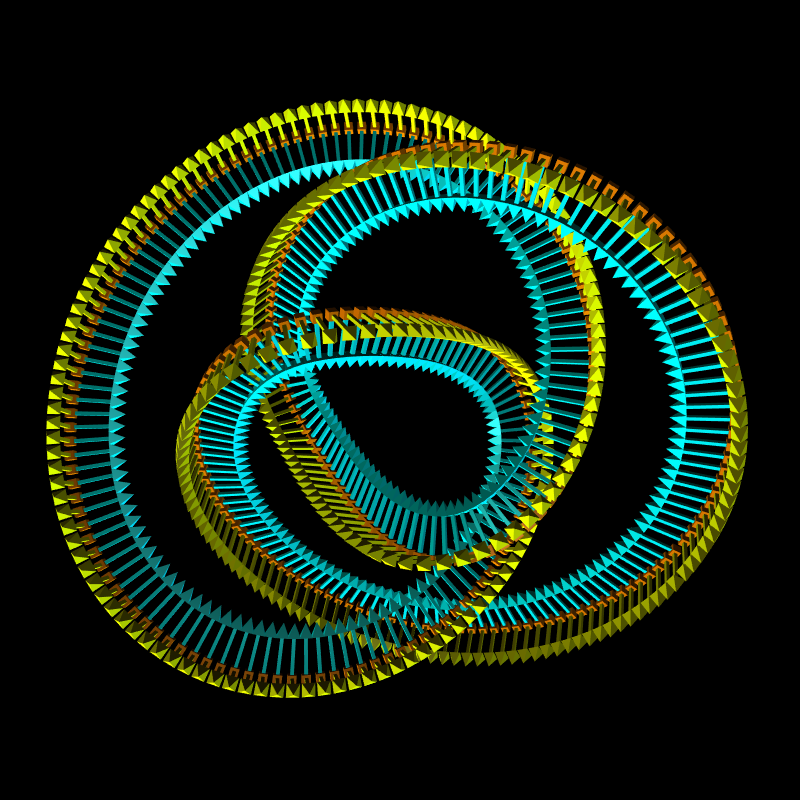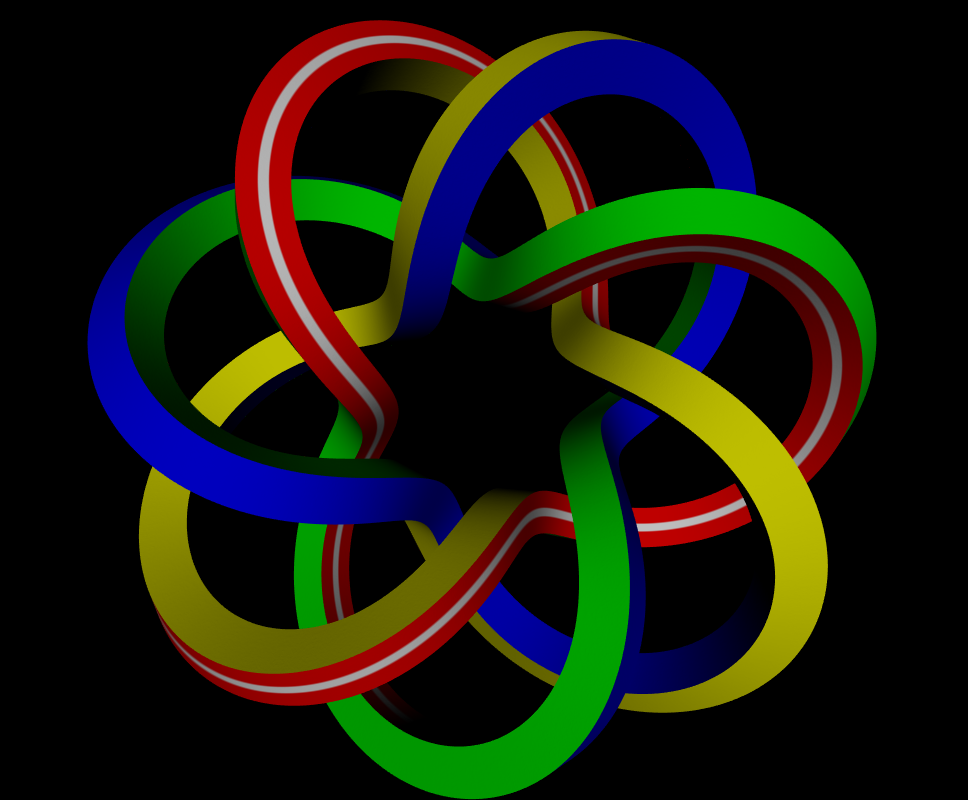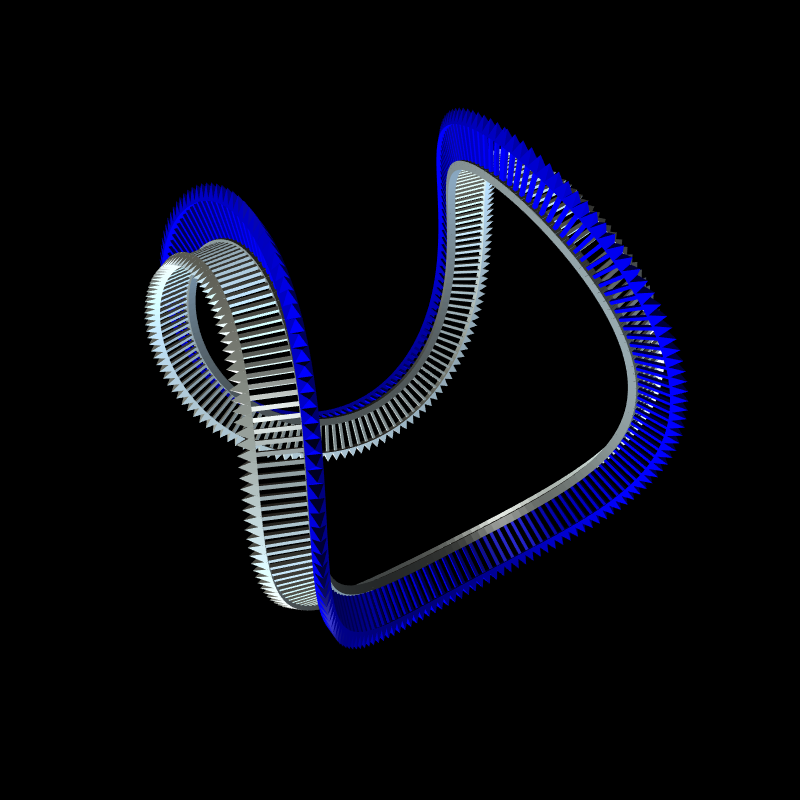# Double reflection method

The double reflection method provides a simple means for approximating the rotation minimizing frame (RMF) of an arbitrary curve. More specifically, it discretely calculates the exact RMF of a curve that is itself an approximation of an arbitrary input curve.

## Rotation minimizing frames

Imagine you have a curve in R3 and that you want to sweep a contour along the curve's path to form a surface. In order to accomplish this, we need to walk along the curve at discrete sampling points and somehow calculate a frame at every sample point. A frame in R3 is composed of a displacement vector x and a basis (t,r,c). The t vector is simply the tangent vector, which we can calculate directly from the curve. We can calculate c as the cross product between tangent vector t and reference vector r. But we have a circle of choices for r. For the most pleasing shape, we want to select r such that the set of frames exhibits no instantaneous twisting about the tangent t. This frame is referred to as the rotation minimizing frame (RMF). The RMF can be described as a differential equation, but this would require using numerical methods to approximate it which tend to be very slow and numerically unstable. If we're willing to accept an approximation of the RMF, then we can use the double reflection method to achieve a high-quality result with very few calculations.

## Details

The double reflection method is deceptively simple. It calculates frames iteratively. Given a frame with displacement xi and basis (ti,ri,ci), and given the next frame's displacement xi+1 and tangent ti+1, the reference vector ri+1 is calculated as:It should be clear by now why this method is named the double reflection method. At the risk of going a little too deep, combining two reflections actually encodes a rotation. So in effect, each subsequent basis is a rotation of the basis that came immediately before it. This method has a ton of beautiful invariance properties and is asymptotically optimal, while being very compact and efficient. I tend to believe this method can even be generalized to any number of dimensions: simply apply the double reflection to each reference vector. In fact, we need not calculate the c vector as the cross product between t and r: we can just carry it forward with the double reflection! Something of note is that other methods tend to blow up when the curve is a straight line; interestingly, the double reflection method couldn't be more stable when the curve is a straight line.

Because the rotation minimizing frame is completely specified by the tangent vectors alone, it is useful to have a formulation of the double reflection method that does not depend on the displacements. Additionally, the original listing suffers from numerical instability when the points are sampled too densely. Using the Euler-Maclaurin integration formula, we can rewrite v1 without sacrificing fourth-order accuracy:## Visualization

As I love visualizing complex concepts, I built a program from scratch using C++ and Direct3D. The program allows you to supply a curve displacement function and optional velocity function, and it will sample the displacements and velocities along the curve. This data is fed into the double reflection method, which outputs the reference vector portion of the basis vectors. The third basis vector is trivially calculated as the cross product between the tangent and reference vectors. The contour shape is unrestricted and is also programmable. I wrote a wide range of shaders, including car paint and Oren-Nayar shaders. The program also lets you visualize the basis vectors themselves.